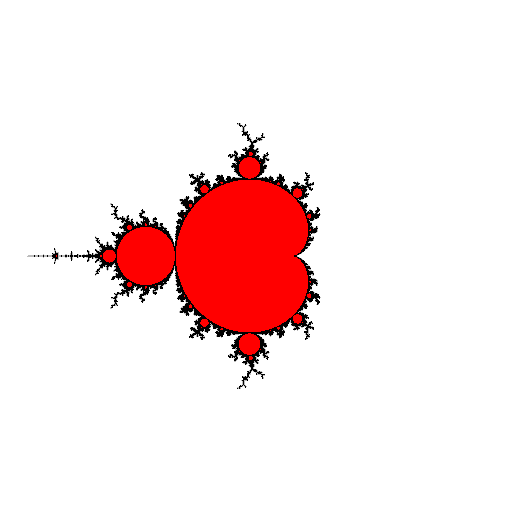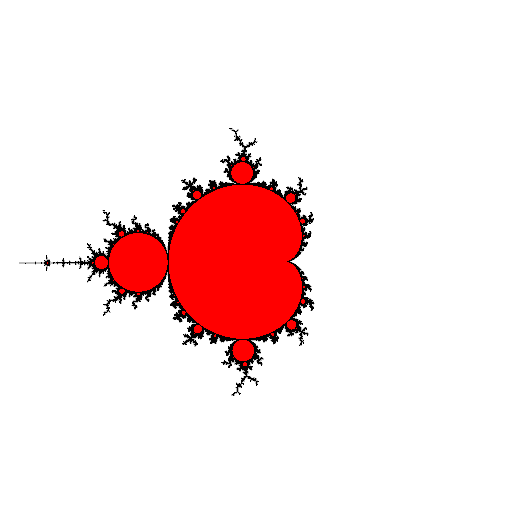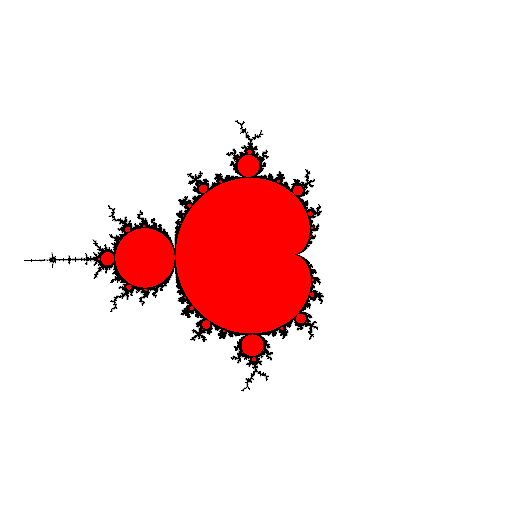# mathr / blog / #

## Approximate self-similarity

The Mandelbrot set is approximately self-similar, containing miniature baby Mandelbrot set copies. However, all of these copies are distorted, because there is only one perfect circle in the Mandelbrot set. The complex-valued size estimate can be used as a multiplier for looping zoom animations, though the difference in decorations and visible distortion make the seam a little jarring. Here are some examples:period $$3$$ near $$-2$$period $$4$$ near $$i$$period $$5$$ near $$-1.5 + 0.5 i$$

The trick to the looping zoom is to find an appropriate center: if the nucleus of the baby is $$c$$ and the complex size is $$r$$, there is another miniature copy near the baby around $$c + r c$$ with size approximately $$r^2$$. Taking the limit gives a geometric progression:

$c + r c + r^2 c + \cdots = \frac{c}{1 - r}$

Here's the code used to render the images (also found in the mandelbrot-graphics repository):

#include <stdio.h>
#include <mandelbrot-graphics.h>

int main(int argc, char **argv) {
(void) argc;
(void) argv;
const double _Complex r0 = 1;
const double _Complex c0 = 0;
int periods = { 3, 4, 5 };
double _Complex c1s = { -2, I, -1.5 + I * 0.5 };
int w = 512;
int h = 512;
m_pixel_t red   = m_pixel_rgba(1, 0, 0, 1);
m_pixel_t black = m_pixel_rgba(0, 0, 0, 1);
m_pixel_t white = m_pixel_rgba(1, 1, 1, 1);
double er = 600;
int maxiters = 1000;
m_image *image = m_image_new(w, h);
if (image) {
m_d_colour_t *colour = m_d_colour_minimal(red, black, white);
if (colour) {
for (int k = 0; k < 3; ++k) {
int period = periods[k];
double _Complex c1 = c1s[k];
m_d_nucleus(&c1, c1, period, 64);
double _Complex r1 = m_d_size(c1, period);
for (int frame = 0; frame < 50; ++frame) {
double f = (frame + 0.5) / 50;
double _Complex r = cpow((r1), f) * cpow((r0), 1 - f);
double _Complex c = c1 / (1 - r1);
m_d_transform *rect = m_d_transform_rectangular(w, h, 0, 1);
m_d_transform *move1 = m_d_transform_linear(- c / 2.25, 1);
m_d_transform *zoom = m_d_transform_linear(0, r * 2.25);
m_d_transform *move2 = m_d_transform_linear(c, 1);
m_d_transform *rm1 = m_d_transform_compose(rect, move1);
m_d_transform *zm2 = m_d_transform_compose(zoom, move2);
m_d_transform *transform = m_d_transform_compose(rm1, zm2);
m_d_render_scanline(image, transform, er, maxiters, colour);
char filename;
snprintf(filename, 100, "%d-%02d.png", k, frame);
m_image_save_png(image, filename);
m_d_transform_delete(transform);
m_d_transform_delete(zm2);
m_d_transform_delete(rm1);
m_d_transform_delete(move2);
m_d_transform_delete(zoom);
m_d_transform_delete(move1);
m_d_transform_delete(rect);
}
}
m_d_colour_delete(colour);
}
m_image_delete(image);
}
return 0;
}

I used ImageMagick to convert each PNG to GIF, then gifsicle to combine into animations.